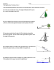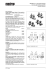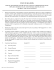# ENS1101 - Engineering Mechanics Assignment Lecturer: Dr Shuo LI

## Transcription

ENS1101 - Engineering Mechanics Assignment Lecturer: Dr Shuo LI
```ENS1101 - Engineering Mechanics
Assignment
Lecturer: Dr Shuo LI
Instructions:

Students need to work in groups of 2 to 3 and submit one assignment per group.

Students need to attach a cover sheet with the names and IDs of each participant.

Do not use plastic folders, simply staple the top left corner of your assignment.

Submit the assignment to the engineering front office in building 23 by 24/10/2014, 3
pm, penalties will apply to late submissions. Do not email me the assignment!

Don’t forget to draw free body diagrams when calculating forces and moments.

Don’t forget to label lengths and angles when doing geometric calculations.

Don’t forget to include your axes and units when plotting graphs.

Show all steps in your solution and state your assumptions, it is better to include an
extra step than not.

Marks will be deducted for sloppy solutions and diagrams!

This assignment will contribute to 15% of the overall mark for the unit.
Good luck!
1 1. The ramp shown below is used to assist passengers boarding light commuter
aircrafts. The average mass of the ramp and passengers is 800 kg with the center of
mass located at point G. As the ramp angle θ increases, the hydraulic cylinder
extends but the distance between the center of mass G and pivot C remains fixed.
(10 points)
a) Derive the equation for length AB as a function of BC, AC and θ. (1 points)
b) Derive the equation for angle BAC as a function of BC, AB and θ. (2 points
c) Calculate the pivot reaction force (FC) and hydraulic cylinder force (FAB) at a
ramp angle of 10 degrees. (4 points)
d) Plot the variation of Fc and FAB as a function of θ over the range 10 < θ < 20.
(3 points)
2 2. Consider the plane truss shown below. The reaction force at points A and B act at
an angle of α = 30 and β = 22 degrees relative to the horizontal axis. (10 points)
a) Determine the reaction forces at points A and B. (2 points)
b) Determine the force in member AH. (2 marks)
c) Determine the force in members BH and BC. (2 points)
d) Determine the force in members CH and HG. (2 points)
e) Determine the force in members CD and CG. (2 points)
3 3. The pipe clamp ends are designed to fit a standard 20 mm steel water pipe. The
right hand fitting can slide along the pipe when handle C is held up to release its grip
on the pipe. When the handle is released and a clamping load of F = 1000 N is
applied by the screw, the fitting makes contact with the pipe at points A and B. In this
position, the spring is compressed with a spring constant of 40 N/mm. Assume that
the handle is a frictionless surface.(10 points)
a) Determine the static friction coefficient at point B needed to avoid slip under
b) Calculate the force acting at point A and B under loading. (2 points)
d) Calculate the handle force required to loosen the fitting. Assume that OC
remains parallel to the pipe. (2 points)
e) Calculate the force supported by pin O when the above handle force is
applied. (2 points)
End of assignment
4 ```

### installation of an angle board * * * thermopian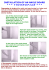### SAMPLE PROBLEMS### Fernco Flexible Coupling Installation - Fernco### What is the difference between Pipe and Round Tube?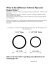### Hymax Couplings 1.5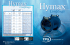### Tugas Analisa Numerik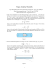### SunPipe - Urecon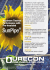### Data Sheet - Carlton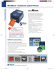### HEA TER TEMPLA TE PROP END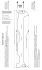### FR Pipe MEGA www.marleypipesystems.co.za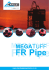### ES-225 for Sewerage Assets™ A4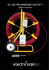### Pipemaster Flyer Front (no contact) 9_3_08### ProTek Composite Pipe Shoe Information Sheet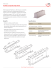### lfucg follows kytc lead and approves ads and hancor hdpe pipe for### LOKRING PIPE SOLUTIONS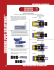### P. LeClair - The University of Alabama### Inverse Trig Functions You know how to find the missing side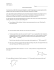### CE 40450 Hydraulics, Spring 2015, Homework 5 Due Date### AC Geo/Adv.Alg Unit 1 Worksheet 11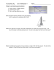### Trig Applications ws day 2 geo 14